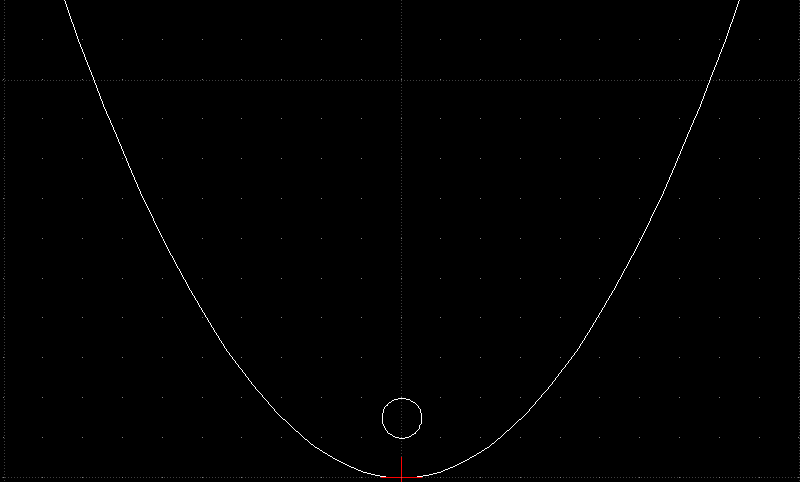Zach needed a parabola in QCAD, so I whipped up a quick script to generate DXF parabolas.  Source inside.

You’ll need the SDXF dxf generator library.  You can get it here. Just dump it in the same directory as this script, and you’re good to go.  Twiddle the parameters, run the script, and you’ve got your parabola.

```import sdxf

# Set your parabola's parameters here
focus=1.5
range=(-10.0,10.0)
delta=0.2

d=sdxf.Drawing()

d.layers.append(sdxf.Layer(name="PARABOLA"))

def parabolaPoint(x,focus):
return (x,(x**2)/(4*focus))

middle = sum(range)/2.0
if (middle - range < delta):
p1 = parabolaPoint(range,focus)
p2 = parabolaPoint(range,focus)
drawing.append(sdxf.Line(points=[p1,p2],layer="PARABOLA"))
else: